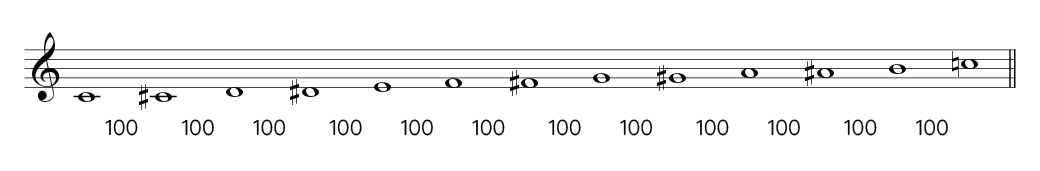#### From logarithms to cents

In the previous section, we almost got to Ellis's formula for calculating cents. Here we will follow the process in more detail and arrive at the final formula.

Let's find the base 2 logarithms of the frequencies of two notes (C4 and C5) at the distance of one octave:

Note Frequency Logarithm base 2 Power
C4 260 Hz. log2 (260) = 8.022367813028454 28.022367813028454 = 260
C5 520 Hz. log2 (520) = 9.022367813028454 29.022367813028454 = 520

#### How do we calculate logarithms base 2?

Your calculator probably does not have the option of calculating base 2 logarithms. Programs like Excel, Numbers or Google Sheets can do it with the following formula:

=log(value, base)

where value is the number you want to get the logarithm of and base is the base you want to use (2 in our case).

Or you can use our calculator:

If we subtract the numbers of the base 2 logarithms of the frequencies of C4 and C5 we obtain:

9.022367813028454 - 8.022367813028454 = 1

The difference between the base 2 logarithms of the frequencies of the notes of an octave results in 1 (if they were two octaves, the result would be 2). Ellis proposes multiplying this number by 1200 to obtain the cents. Why 1200? Because the octave is divided into 12 notes and 1200 assigns 100 cents to each semitone.We need to multiply by 1200 in our formula:

$cents = (log_2(f_2) - log_2(f_1)) * 1200$

Note: You must subtract the logarithms first and then multiply by 1200.